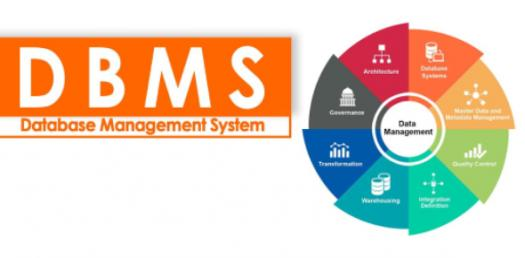# Degree Of Relationship! DBMS Trivia Quiz

10 Questions | Total Attempts: 53Settings.

Related Topics
• 1.
There is 4 degrees of relationships.
• A.

TRUE

• B.

FALSE

• 2.
Cardinality constraints are the number of an entity in one instance that can or must be associated with each instance of another entity.
• A.

TRUE

• B.

FALSE

• 3.
The relationship is an association among the instances of one or more entity types that are not of interest to the organization.
• A.

TRUE

• B.

FALSE

• 4.
Relationship instance is a meaningful association between (or among) entity type.
• A.

TRUE

• B.

FALSE

• 5.
A ternary relationship is a simultaneous relationship between the instance of 2-3 entity types.
• A.

TRUE

• B.

FALSE

• 6.
Entity is a verb and relationship is a noun.
• A.

TRUE

• B.

FALSE

• 7.
Degree is the number of entity types that participate in a relationship.
• A.

TRUE

• B.

FALSE

• 8.
Minimum Cardinality is the minimum number of instances of one entity that may be associated with each instance of another entity.
• A.

TRUE

• B.

FALSE

• 9.
Maximum cardinality is the maximum number of instances of one entity that may be associated with each instance of another entity.
• A.

TRUE

• B.

FALSE

• 10.
The unary relationship is the relationship on itself.
• A.

TRUE

• B.

FALSE